# 概述

Excel固然功能强大，也有许多函数实现数据处理功能，但是Excel仍需大量人工操作，虽然能嵌入VB脚本宏，但也容易染上宏病毒。python作为解释性语言，在数据处理方面拥有强大的函数库以及第三方库，excel作为主要基础数据源之一，在利用数据进行分析前往往需要预先对数据进行整理。因此，本文就python处理excel数据进行了学习，主要分为python对excel数据处理的常用数据类型以及常用函数，用python语言实现excel基本操作。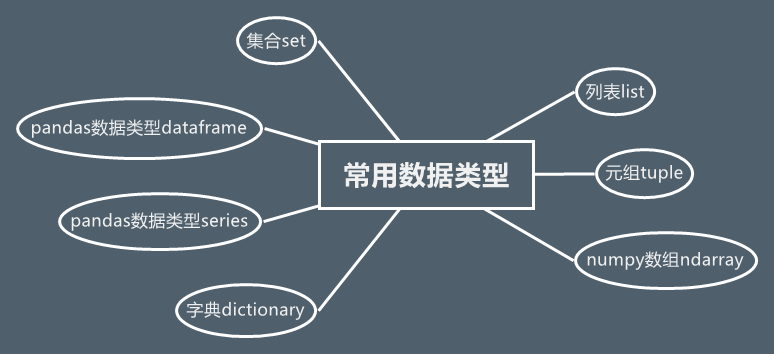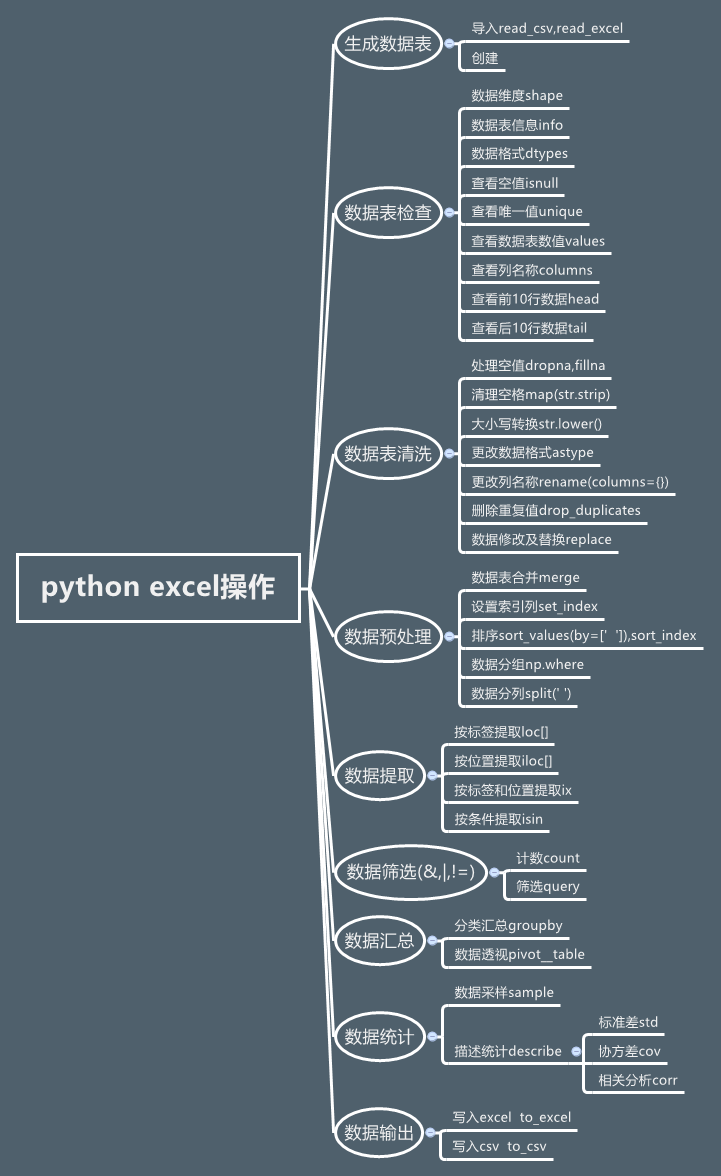# 常用数据类型序列sequence, 列表list, 元组tuple, array,字典dictionary，series,dataframe,集合set区别

• 索引
• 切片
• 成员检查
• 计算序列的长度
• 取序列中的最大、最小值

## 列表list

### 概述用途

• 一组有序项目的集合。可变的数据类型【可进行增删改查】
• 列表是以方括号“[]”包围的数据集合，不同成员以“,”分隔。
• 列表中可以包含任何数据类型，也可包含另一个列表
• 列表可通过序号访问其中成员

### 声明方式

example = [0,1,2,3,4,5,6,7,8,9]


### API

#### Python列表脚本操作符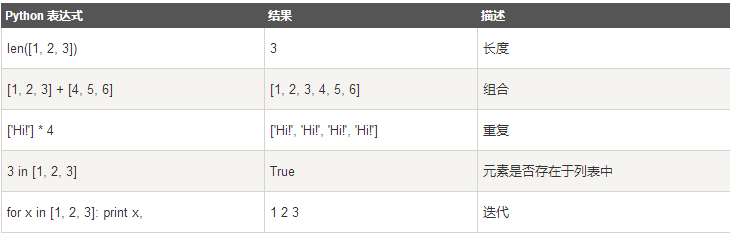#### Python列表函数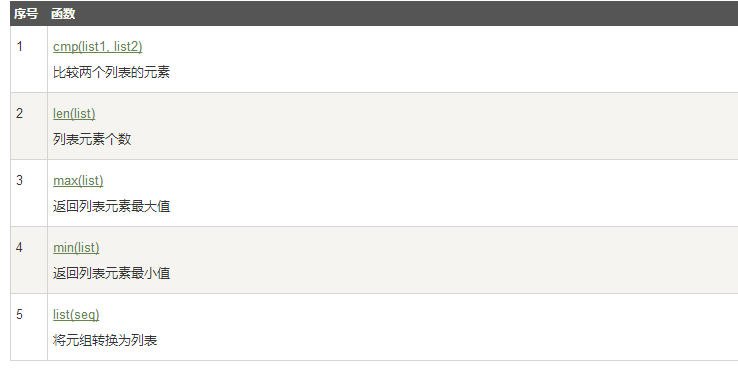#### Python列表方法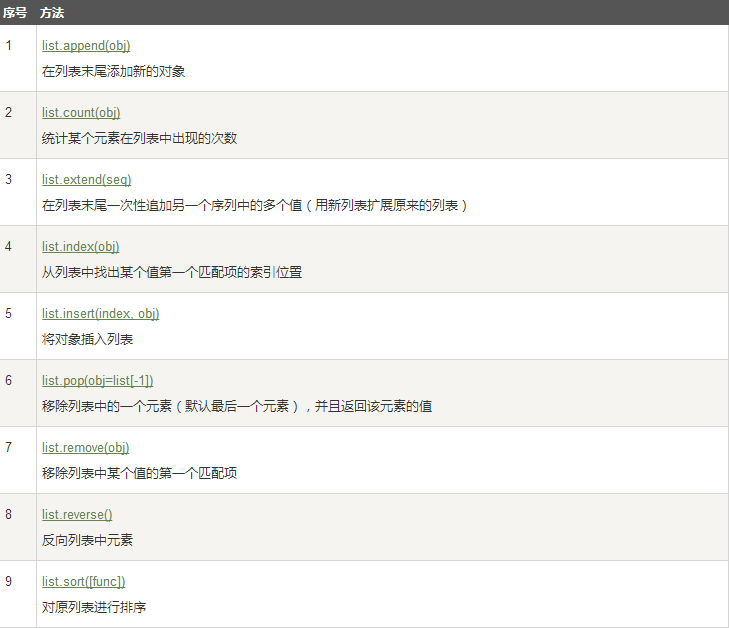#### 切片

#列表操作补充--切片操作
example = [0,1,2,3,4,5,6,7,8,9]
#打印某一区间 左闭右开
print(example[4:8])
#想包含最后一个
print(example[4:])
#包含首个
print(example[:8])
#所有的
print(example[:])
#第三个参数为步长
print(example[1:8:2])
#倒序输出
print(example[::-1])
#列表合并
a = [1,2,3]
b = [4,5,6]
print(a+b)
#替换
ex = [1,2,3,4,5,6]
ex[4:]=[9,8,7]
print(ex)#将56换为987


#### 判断列表中

>>> list4=[123,["das","aaa"],234]
>>> list4
>>> "aaa" in list4 #in只能判断一个层次的元素
False
>>> "aaa" in list4 #选中列表中的列表进行判断
True
>>> list4
'aaa'


## 元组tuple

### 概述用途

• 不可变序列
• 元组是以圆括号“()”包围的数据集合，不同成员以“,”分隔
• 与列表不同：元组中数据一旦确立就不能改变
• 通过下标进行访问

### 声明方式

L=(1,2,3)



### 元组操作

>>> o =('a','b','c',('d1','d2'))
>>> print o
>>> print o
a
d1

>>> age=22
>>> name='sandra'
>>> print'%s is %d years old'%(name,age)
sandra is 22 years old


（元组中的元素值是不允许修改的，但我们可以对元组进行连接组合，如下实例）

tup1 = (12, 34.56);
tup2 = ('abc', 'xyz');
# 以下修改元组元素操作是非法的。
# tup1 = 100;
# 创建一个新的元组
tup3 = tup1 + tup2;
print tup3;


#通过切片方法添加
a=(1,2,3,4,5,6)
a=a[:2]+(10,)+a[2:]
a


del tup1



### API

#### 元组运算符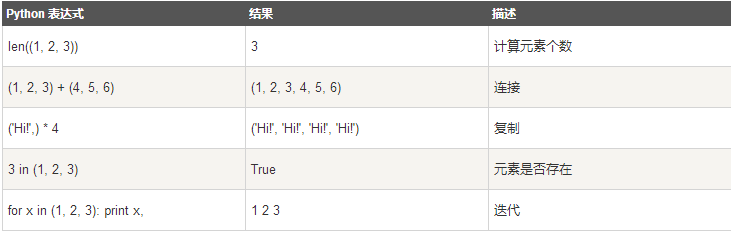#### 元组内置函数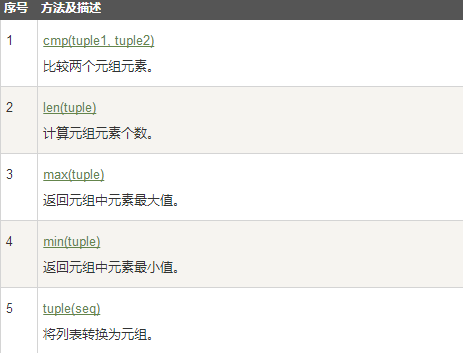## 字典dictionary

### 概述用途

• 字典是另一种可变容器模型，且可存储任意类型对象。

• 字典的每个键值(key=>value)对用冒号(:)分割，每个对之间用逗号(,)分割，整个字典包括在花括号({})中

### 声明方式

d = {key1 : value1, key2 : value2 }
//键必须是唯一的，但值则不必。
//值可以取任何数据类型，但键必须是不可变的，如字符串，数字或元组。


### 字典操作

dict = {'Name': 'Zara', 'Age': 7, 'Class': 'First'};
dict['Name']
dict['Age']


dict = {'Name': 'Zara', 'Age': 7, 'Class': 'First'};
dict['Age'] = 8;
dict['School'] = "DPS School"


dict = {'Name': 'Zara', 'Age': 7, 'Class': 'First'};
del dict['Name']; # 删除键是'Name'的条目
dict.clear();     # 清空词典所有条目
del dict ;        # 删除词典



### API

#### 字典内置函数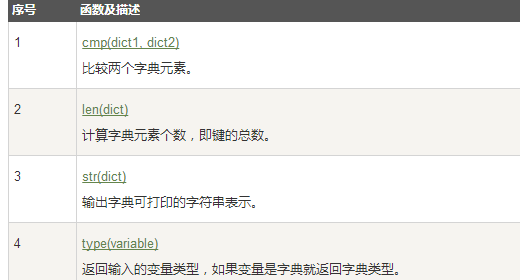#### 字典内置方法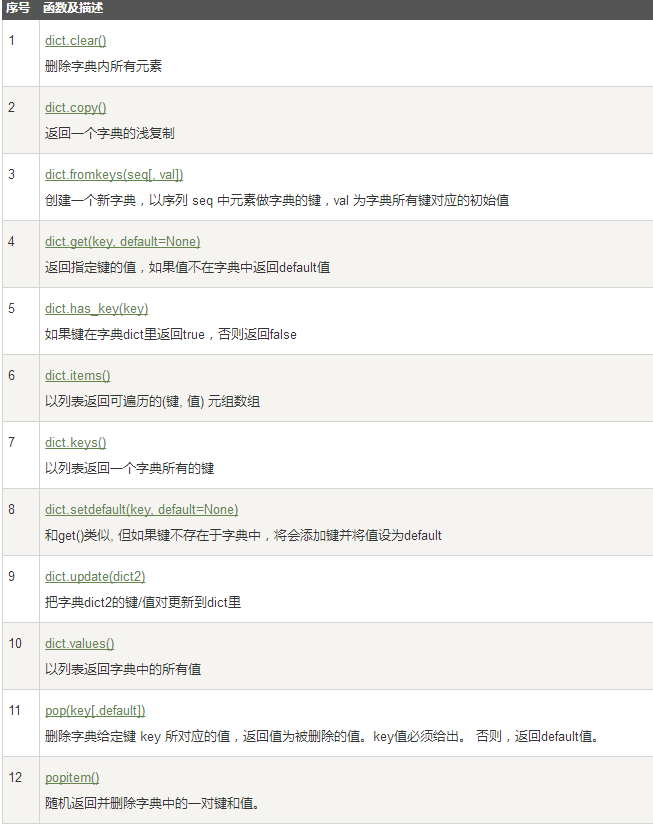## ndarray（数组）–numpy

### 概述用途

python中的list是python的内置数据类型，list中的数据类不必相同的，而array的中的类型必须全部相同。在list中的数据类型保存的是数据的存放的地址，简单的说就是指针，并非数据，这样保存一个list就太麻烦了，例如list1=[1,2,3,‘a’]需要4个指针和四个数据，增加了存储和消耗cpu。numpy中封装的array有很强大的功能，里面存放的都是相同的数据类型
1）numpy array 必须有相同数据类型属性 ，Python list可以是多种数据类型的混合
2）numpy array有一些方便的函数
3）numpy array数组可以是多维的

### 声明方式

np.array用来创建ndarray类型

b=np.array([[1,2,3],[4,5,6],[7,8,9],[10,11,12]])


## series-pandas

### 概述用途

class pandas.Series（data = None，index = None，dtype = None，name = None，copy = False，fastpath = False ）

Series（+， - ，/ , *）之间的操作根据其关联的索引值对齐值 - 它们不必是相同的长度。结果索引将是两个索引的排序并集。

### 声明

Python列表，index与列表元素个数一致


In : import pandas as pd
​
In : list_a = [2,4,5,6]
​
In : pd.Series(list_a)
Out:
0    2
1    4
2    5
3    6
dtype: int64



In : pd.Series(1,index = [1,2,3])
Out:
1    1
2    1
3    1
dtype: int64


Python字典，键值对中的“键”是索引，index从字典中进行选择操作

In : pd.Series({'a':1,'b':3})
Out:
a 1
b 3
dtype: int64
#如果定义的index在原字典中已经存在，那么该索引会一直对应原字典的值，如果index对应不到原字典的值，则会返回NaN
In : pd.Series({'a':1,'b':3},index = ['b','a','c'])
Out:
b 3.0
a 1.0
c NaN
dtype: float64


ndarray，索引和数据都可以通过ndarray类型创建

In : list_b = np.arange(6)
​
In : pd.Series(list_b)
Out:
0    0
1    1
2    2
3    3
4    4
5    5
dtype: int32


In : pd.Series(range(3))
Out:
0    0
1    1
2    2
dtype: int32


### series操作

Series类型包括index和values两部分

In : a = pd.Series({'a':1,'b':5})
In : a.index
Out: Index(['a', 'b'], dtype='object')

In : a.values #返回一个多维数组numpy对象
Out: array([1, 5], dtype=int64)


Series类型的操作类似ndarray类型

#自动索引和自定义索引并存，但不能混用
In : a #自动索引
Out: 1
#自定义索引
In : a['a']
Out: 1
#不能混用
In : a[['a',1]]
Out:
a 1.0
1 NaN
dtype: float64


Series类型的操作类似Python字典类型

#通过自定义索引访问
#对索引保留字in操作，值不可以
In : 'a' in a
Out: True
​
In : 1 in a
Out: False


Series类型在运算中会自动对齐不同索引的数据

In : a = pd.Series([1,3,5],index = ['a','b','c'])
In : b = pd.Series([2,4,5,6],index = ['c,','d','e','b'])
In : a+b
Out:
a NaN
b 9.0
c NaN
c, NaN
d NaN
e NaN


Series对象可以随时修改并即刻生效

In : a.index = ['c','d','e']
In : a
Out:
c 1
d 3
e 5
dtype: int64
In : a+b
Out:
b NaN
c NaN
c, NaN
d 7.0
e 10.0
dtype: float64


## dataframe-pandas

### 概述用途

DataFrame提供的是一个类似表的结构，由多个Series组成，而Series在DataFrame中叫columns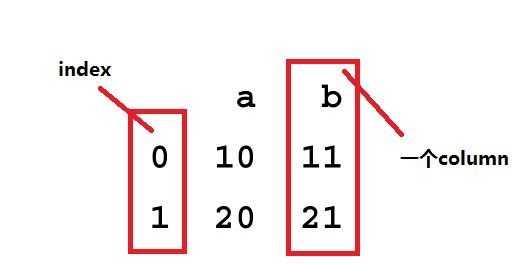### 声明方式

import pandas as pd
from pandas import Series, DataFrame
import numpy as np
data = DataFrame(np.arange(15).reshape(3,5),index=['one','two','three'],columns=['a','b','c','d','e'])
print data

        a   b   c   d   e
one     0   1   2   3   4
two     5   6   7   8   9
three  10  11  12  13  14


#### array

import pandas as pd
import numpy as np
s1=np.array([1,2,3,4])
s2=np.array([5,6,7,8])
df=pd.DataFrame([s1,s2])
print df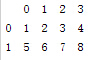#### series列表（效果与二维array相同）

import pandas as pd
import numpy as np
s1=pd.Series(np.array([1,2,3,4]))
s2=pd.Series(np.array([5,6,7,8]))
df=pd.DataFrame([s1,s2])
print df#### value为Series的字典结构

import pandas as pd
import numpy as np

s1=pd.Series(np.array([1,2,3,4]))
s2=pd.Series(np.array([5,6,7,8]))
df=pd.DataFrame({"a":s1,"b":s2});
print df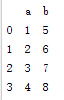### 操作

df=pd.DataFrame({"A":[1,2,3,4],"B":[5,6,7,8],"C":[1,1,1,1]})
df=df[df.A>=2]
print df


df=pd.DataFrame({"A":[1,2,3,4],"B":[5,6,7,8],"C":[1,1,1,1]})
df=df.loc[df.A>2]
print df


.ix[]索引

print df.columns.size#列数 2
print df.iloc[:,0].size#行数 3
print df.ix[].index.values#索引值 0
print df.ix[].values#第一行第一列的值 11
print df.ix[].values#第二行第二列的值 121


### API

group(groupby 形成group)

df = pd.DataFrame({'animal': 'cat dog cat fish dog cat cat'.split(),
'size': list('SSMMMLL'),
'weight': [8, 10, 11, 1, 20, 12, 12],
'adult' : [False] * 5 + [True] * 2});
#列出动物中weight最大的对应size
group=df.groupby("animal").apply(lambda subf: subf['size'][subf['weight'].idxmax()])
print group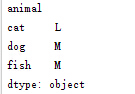df = pd.DataFrame({'animal': 'cat dog cat fish dog cat cat'.split(),
'size': list('SSMMMLL'),
'weight': [8, 10, 11, 1, 20, 12, 12],
'adult' : [False] * 5 + [True] * 2});

group=df.groupby("animal")
cat=group.get_group("cat")
print cat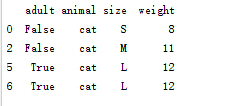## 集合

### 声明

>>> {1,2,"hi",2.23}
{2.23, 2, 'hi', 1}
>>> set("hello")
{'l', 'h', 'e', 'o'}


### 集合操作

>>> a={1,2}
>>> a.update([3,4],[1,2,7])
>>> a
{1, 2, 3, 4, 7}
>>> a.update("hello")
>>> a
{1, 2, 3, 4, 7, 'h', 'e', 'l', 'o'}
>>> a
{1, 2, 3, 4, 'hello', 7, 'h', 'e', 'l', 'o'}


>>> a={1,2,3,4}
>>> a
{2, 3, 4}
>>> a
{2, 3, 4}
>>> a.remove(1)
Traceback (most recent call last):
File "<input>", line 1, in <module>
KeyError: 1


>>> a={3,"a",2.1,1}
>>> a.pop()
>>> a.pop()
>>> a.clear()
>>> a
set()
>>> a.pop()
Traceback (most recent call last):
File "<input>", line 1, in <module>
KeyError: 'pop from an empty set'


### API

#### 集合操作

• 并集：set.union(s),也可以用a|b计算
• 交集：set.intersection(s)，也可以用a&b计算
• 差集：set.difference(s)，也可以用a-b计算
需要注意的是Python提供了一个求对称差集的方法set.symmetric_difference(s)，相当于两个集合互求差集后再求并集，其实就是返回两个集合中只出现一次的元素，也可以用a^b计算。
>>> a={1,2,3,4}
>>> b={3,4,5,6}
>>> a.symmetric_difference(b)
{1, 2, 5, 6}
2
4


set.update(s)操作相当于将两个集合求并集并赋值给原集合，其他几种集合操作也提供各自的update版本来改变原集合的值，形式如intersection_update(),也可以支持多参数形式。

#### 包含关系

• set.isdisjoint(s)：判断两个集合是不是不相交
• set.issuperset(s)：判断集合是不是包含其他集合，等同于a>=b
• set.issubset(s)：判断集合是不是被其他集合包含，等同于a<=b
如果要真包含关系，就用符号操作>和<。

#### 不变集合

Python提供了不能改变元素的集合的实现版本，即不能增加或删除元素，类型名叫frozenset，使用方法如下：

>>> a = frozenset("hello")
>>> a
frozenset({'l', 'h', 'e', 'o'})
2


# python excel操作

##生成数据表

### 准备

import numpy as np
import pandas as pd


### 导入数据表

df=pd.DataFrame(pd.read_csv('name.csv',header=1))


### 创建数据表

df = pd.DataFrame({"id":[1001,1002,1003,1004,1005,1006],
"date":pd.date_range('20130102', periods=6),
"city":['Beijing ', 'SH', ' guangzhou ', 'Shenzhen', 'shanghai', 'BEIJING '],
"age":[23,44,54,32,34,32],
"category":['100-A','100-B','110-A','110-C','210-A','130-F'],
"price":[1200,np.nan,2133,5433,np.nan,4432]},
columns =['id','date','city','category','age','price'])


## 数据表检查

python中处理的数据量通常会比较大，，我们无法一目了然的了解数据表的整体情况，必须要通过一些方法来获得数据表的关键信息。数据表检查的另一个目的是了解数据的概况，例如整个数据表的大小，所占空间，数据格式，是否有空值和重复项和具体的数据内容。为后面的清洗和预处理做好准备。

### 数据维度

#查看数据表的维度
df.shape
(6, 6)


### 数据表信息

#数据表信息
df.info()

&lt;class 'pandas.core.frame.DataFrame'&gt;
RangeIndex: 6 entries, 0 to 5
Data columns (total 6 columns):
id          6 non-null int64
date        6 non-null datetime64[ns]
city        6 non-null object
category    6 non-null object
age         6 non-null int64
price       4 non-null float64
dtypes: datetime64[ns](1), float64(1), int64(2), object(2)
memory usage: 368.0+ bytes


### 查看数据格式

#查看数据表各列格式
df.dtypes

id                   int64
date        datetime64[ns]
city                object
category            object
age                  int64
price              float64
dtype: object

#查看单列格式
df['B'].dtype

dtype('int64')



### 查看空值

Isnull是Python中检验空值的函数，返回的结果是逻辑值，包含空值返回True，不包含则返回False。可以对整个数据表进行检查，也可以单独对某一列进行空值检查。

#检查数据空值
df.isnull()

#检查特定列空值
df['price'].isnull()
0    False
1     True
2    False
3    False
4     True
5    False

Name: price, dtype: bool


### 查看唯一值 （删除重复项）

#查看city列中的唯一值
df['city'].unique()

array(['Beijing ', 'SH', ' guangzhou ', 'Shenzhen', 'shanghai', 'BEIJING '], dtype=object)


### 查看数据表数值

#查看数据表的值
df.values
array([[1001, Timestamp('2013-01-02 00:00:00'), 'Beijing ', '100-A', 23,
1200.0],
[1002, Timestamp('2013-01-03 00:00:00'), 'SH', '100-B', 44, nan],
[1003, Timestamp('2013-01-04 00:00:00'), ' guangzhou ', '110-A', 54,
2133.0],
[1004, Timestamp('2013-01-05 00:00:00'), 'Shenzhen', '110-C', 32,
5433.0],
[1005, Timestamp('2013-01-06 00:00:00'), 'shanghai', '210-A', 34,
nan],
[1006, Timestamp('2013-01-07 00:00:00'), 'BEIJING ', '130-F', 32,
4432.0]], dtype=object)


### 查看列名称

#查看列名称
df.columns
Index(['id', 'date', 'city', 'category', 'age', 'price'], dtype='object')


### 查看前10行数据

#查看前3行数据


### 查看后10行数据

#查看最后3行
df.tail(3)


## 数据表清洗

### 处理空值

#删除数据表中含有空值的行
df.dropna(how='any')


#使用数字0填充数据表中空值
df.fillna(value=0)

#使用数字0填充数据表中空值
df.fillna(value=0)

#使用price均值对NA进行填充
df['price'].fillna(df['price'].mean())
0    1200.0
1    3299.5
2    2133.0
3    5433.0
4    3299.5
5    4432.0
Name: price, dtype: float64


### 清理空格

#清除city字段中的字符空格
df['city']=df['city'].map(str.strip)


### 大小写转换

#city列大小写转换
df['city']=df['city'].str.lower()


### 更改数据格式

#更改数据格式
df['price'].astype('int')
0    1200
1    3299
2    2133
3    5433
4    3299
5    4432
Name: price, dtype: int32


### 更改列名称

#更改列名称
df.rename(columns={'category': 'category-size'})


### 删除重复值

#删除后出现的重复值
df['city'].drop_duplicates()
0      beijing
1      sh
2      guangzhou
3     shenzhen
4     shanghai
Name: city, dtype: object

#删除先出现的重复值
df['city'].drop_duplicates(keep='last')
1      sh
2      guangzhou
3     shenzhen
4     shanghai
5     beijing
Name: city, dtype: objec


### 数值修改及替换

#数据替换
df['city'].replace('sh', 'shanghai')


## 数据预处理

### 数据表合并

#数据表匹配合并，inner模式
df_inner=pd.merge(df,df1,how='inner')
#其他数据表匹配模式
df_left=pd.merge(df,df1,how='left')
df_right=pd.merge(df,df1,how='right')
df_outer=pd.merge(df,df1,how='outer')


### 设置索引列

#设置索引列
df_inner.set_index('id')


### 排序（按索引，按数值）

#按特定列的值排序
df_inner.sort_values(by=['age'])

#按索引列排序
df_inner.sort_index()


### 数据分组（vlookup）

Excel中可以通过VLOOKUP函数进行近似匹配来完成对数值的分组，或者使用“数据透视表”来完成分组。相应的 python中使用where函数完成数据分组。

#如果price列的值>3000，group列显示high，否则显示low
df_inner['group'] = np.where(df_inner['price'] > 3000,'high','low')

#对复合多个条件的数据进行分组标记
df_inner.loc[(df_inner['city'] == 'beijing') & (df_inner['price'] >= 4000), 'sign']=1


### 数据分列（对字段拆分）

#对category字段的值依次进行分列，并创建数据表，索引值为df_inner的索引列，列名称为category和size
pd.DataFrame((x.split('-') for x in df_inner['category']),index=df_inner.index,columns=['category','size'])

#将完成分列后的数据表与原df_inner数据表进行匹配
df_inner=pd.merge(df_inner,split,right_index=True, left_index=True)


## 数据提取

### 按标签提取(loc)

#按索引提取单行的数值
df_inner.loc
id 1004
date 2013-01-05 00:00:00
city shenzhen
category 110-C
age 32
price 5433
gender female
m-point 40
pay Y
group high
sign NaN
category_1 110
size C
Name: 3, dtype: object


#按索引提取区域行数值
df_inner.loc[0:5]


Reset_index函数用于恢复索引，这里我们重新将date字段的日期设置为数据表的索引，并按日期进行数据提取。

#重设索引
df_inner.reset_index()

#设置日期为索引
df_inner=df_inner.set_index('date')


#提取4日之前的所有数据
df_inner[:'2013-01-04']


### 按位置提取(iloc)

#使用iloc按位置区域提取数据
df_inner.iloc[:3,:2]


iloc函数除了可以按区域提取数据，还可以按位置逐条提取，前面方括号中的0,2,5表示数据所在行的位置，后面方括号中的数表示所在列的位置。

#使用iloc按位置单独提取数据
df_inner.iloc[[0,2,5],[4,5]]


### 按标签和位置提取(ix)

ix是loc和iloc的混合，既能按索引标签提取，也能按位置进行数据提取。下面代码中行的位置按索引日期设置，列按位置设置。

#使用ix按索引标签和位置混合提取数据
df_inner.ix[:'2013-01-03',:4]


### 按条件提取(区域和条件值)

#判断city列的值是否为beijing
df_inner['city'].isin(['beijing'])

date
2013-01-02 True
2013-01-05 False
2013-01-07 True
2013-01-06 False
2013-01-03 False
2013-01-04 False
Name: city, dtype: bool


#先判断city列里是否包含beijing和shanghai，然后将复合条件的数据提取出来。
df_inner.loc[df_inner['city'].isin(['beijing','shanghai'])]


category=df_inner['category']
0 100-A
3 110-C
5 130-F
4 210-A
1 100-B
2 110-A
Name: category, dtype: object

#提取前三个字符，并生成数据表
pd.DataFrame(category.str[:3])


## 数据筛选

### 按条件筛选（与，或，非）

#使用“与”条件进行筛选
df_inner.loc[(df_inner['age'] > 25) & (df_inner['city'] == 'beijing'), ['id','city','age','category','gender']]

#使用“或”条件筛选
df_inner.loc[(df_inner['age'] > 25) | (df_inner['city'] == 'beijing'), ['id','city','age','category','gender']].sort(['age'])


#对筛选后的数据按price字段进行求和
df_inner.loc[(df_inner['age'] > 25) | (df_inner['city'] == 'beijing'),
['id','city','age','category','gender','price']].sort(['age']).price.sum()

19796

#使用“非”条件进行筛选
df_inner.loc[(df_inner['city'] != 'beijing'), ['id','city','age','category','gender']].sort(['id'])


#对筛选后的数据按city列进行计数
df_inner.loc[(df_inner['city'] != 'beijing'), ['id','city','age','category','gender']].sort(['id']).city.count()
4


#使用query函数进行筛选
df_inner.query('city == ["beijing", "shanghai"]')


#对筛选后的结果按price进行求和
df_inner.query('city == ["beijing", "shanghai"]').price.sum()
12230


## 数据汇总

Excel中使用分类汇总和数据透视可以按特定维度对数据进行汇总，python中使用的主要函数是groupby和pivot_table。下面分别介绍这两个函数的使用方法。

### 分类汇总

Groupby是进行分类汇总的函数，使用方法很简单，制定要分组的列名称就可以，也可以同时制定多个列名称，groupby按列名称出现的顺序进行分组。同时要制定分组后的汇总方式，常见的是计数和求和两种。

#对所有列进行计数汇总
df_inner.groupby('city').count()

#对特定的ID列进行计数汇总
df_inner.groupby('city')['id'].count()
city
beijing 2
guangzhou 1
shanghai 2
shenzhen 1
Name: id, dtype: int64

#对两个字段进行汇总计数
df_inner.groupby(['city','size'])['id'].count()
city size
beijing A 1
F 1
guangzhou A 1
shanghai A 1
B 1
shenzhen C 1
Name: id, dtype: int64


#对city字段进行汇总并计算price的合计和均值。
df_inner.groupby('city')['price'].agg([len,np.sum, np.mean])


### 数据透视

Excel中的插入目录下提供“数据透视表”功能对数据表按特定维度进行汇总。Python中也提供了数据透视表功能。通过pivot_table函数实现同样的效果。

#数据透视表
pd.pivot_table(df_inner,index=["city"],values=["price"],columns=["size"],aggfunc=[len,np.sum],fill_value=0,margins=True)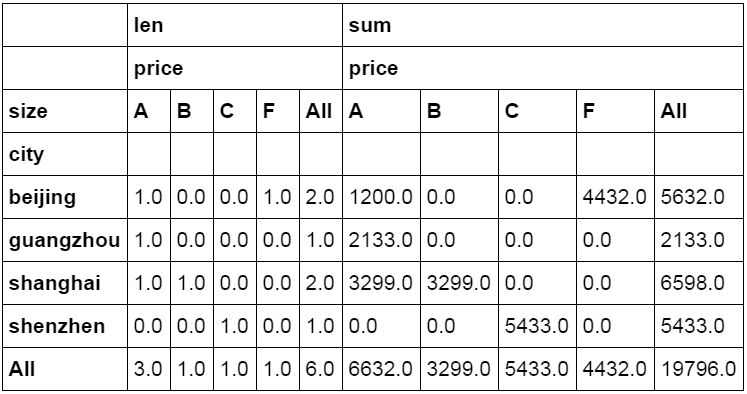## 数据统计

### 数据采样

Python通过sample函数完成数据采样。

#简单的数据采样
df_inner.sample(n=3)


Weights参数是采样的权重，通过设置不同的权重可以更改采样的结果，权重高的数据将更有希望被选中。这里手动设置6条数据的权重值。将前面4个设置为0，后面两个分别设置为0.5。

#手动设置采样权重
weights = [0, 0, 0, 0, 0.5, 0.5]
f_inner.sample(n=2, weights=weights)

#采样后不放回
df_inner.sample(n=6, replace=False)

#采样后放回
df_inner.sample(n=6, replace=True)


### 描述统计

Python中可以通过Describe对数据进行描述统计。
Describe函数是进行描述统计的函数，自动生成数据的数量，均值，标准差等数据。下面的代码中对数据表进行描述统计，并使用round函数设置结果显示的小数位。并对结果数据进行转置。

#数据表描述性统计
df_inner.describe().round(2).T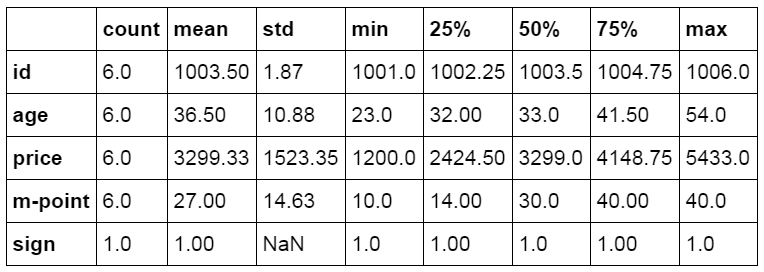#### 标准差

Python中的Std函数用来接算特定数据列的标准差。

#标准差
df_inner['price'].std()
1523.3516556155596


### 协方差

Excel中的数据分析功能中提供协方差的计算，python中通过cov函数计算两个字段或数据表中各字段间的协方差。
Cov函数用来计算两个字段间的协方差，可以只对特定字段进行计算，也可以对整个数据表中各个列之间进行计算。

#两个字段间的协方差
df_inner['price'].cov(df_inner['m-point'])
17263.200000000001

#数据表中所有字段间的协方差
df_inner.cov()


#### 相关分析

Excel的数据分析功能中提供了相关系数的计算功能，python中则通过corr函数完成相关分析的操作，并返回相关系数。
Corr函数用来计算数据间的相关系数，可以单独对特定数据进行计算，也可以对整个数据表中各个列进行计算。相关系数在-1到1之间，接近1为正相关，接近-1为负相关，0为不相关。

#相关性分析
df_inner['price'].corr(df_inner['m-point'])
0.77466555617085264

#数据表相关性分析
df_inner.corr()


## 数据输出

### 写入excel

#输出到excel格式
df_inner.to_excel('excel_to_python.xlsx', sheet_name='bluewhale_cc')


### 写入csv

#输出到CSV格式
df_inner.to_csv('excel_to_python.csv')


#创建数据表
df = pd.DataFrame({"id":[1001,1002,1003,1004,1005,1006],
"date":pd.date_range('20130102', periods=6),
"city":['Beijing ', 'SH', ' guangzhou ', 'Shenzhen', 'shanghai', 'BEIJING '],
"age":[23,44,54,32,34,32],
"category":['100-A','100-B','110-A','110-C','210-A','130-F'],
"price":[1200,np.nan,2133,5433,np.nan,4432]},
columns =['id','date','city','category','age','price'])

#创建自定义函数
def table_info(x):
shape=x.shape
types=x.dtypes
colums=x.columns
print("数据维度(行，列):\n",shape)
print("数据格式:\n",types)
print("列名称:\n",colums)

#调用自定义函数获取df数据表信息并输出结果
table_info(df)

(6, 6)

id int64
date datetime64[ns]
city object
category object
age int64
price float64
dtype: object

Index(['id', 'date', 'city', 'category', 'age', 'price'], dtype='object')


# 好的学习资料

04-26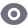3万+

#### python高手之路python处理excel文件(方法汇总)

02-214615

#### 如何用Python增强Excel，减少处理复杂数据的痛苦？04-122万+

#### Python 一个简单的读取excel例子

12-226万+

#### 零基础使用Python读写处理Excel表格

03-062万+

#### Python读取Excel文件

04-212万+

#### 采用python批量统计分析Excel表格数据

07-296955

#### Python数据操作—处理Excel数据

04-194364

#### Python 处理Excel内的数据（案例介绍*2）

07-102万+

#### 如何利用Python编程批量处理Excel来提高日常工作效率!

04-243645

#### python处理excel的一天（使用python做一些处理，excel我是真不怎么会，python也在学，加油）(涉及列表，字典，从文件读取，写入文件操作,一些错误总结）©️2020 CSDN 皮肤主题: 鲸 设计师: meimeiellie点击重新获取扫码支付1.余额是钱包充值的虚拟货币，按照1:1的比例进行支付金额的抵扣。
2.余额无法直接购买下载，可以购买VIP、C币套餐、付费专栏及课程。余额充值